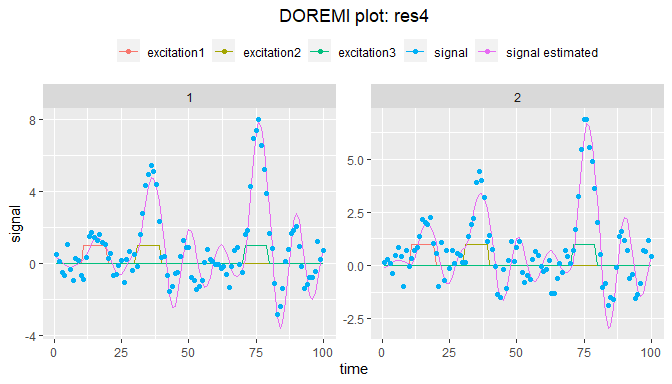# second-order

library(doremi)

# SECOND ORDER DIFFERENTIAL EQUATIONS

The differential equation considered in this case is the following:

$\frac{d^2y}{dt} + 2\xi\omega_{n}\frac{dy}{dt} + \omega_{n}^2 y = k\omega_{n}^2u(t)$ (1)

Where:

• $$y(t)$$ is the signal to be analyzed

• $$\frac{dy}{dt}$$ is its first derivative

• $$\frac{d^2y}{dt}$$ is its second derivative

• $$u(t)$$ is the excitation term perturbing the dynamics of $$y(t)$$

And regarding the coefficients:

• $$\omega_{n} = \frac{2\pi}{T}$$ is the system’s natural frequency, the frequency with which the system would oscillate if there were no damping. $$T$$ is the corresponding period of oscillation. The term $$\omega_{n}^2$$ represents thus the ratio between the attraction to the equilibrium and the inertia. If we considered the example of a mass attached to a spring, this term would represent the ratio of the spring constant and the object’s mass.

• $$\xi$$ is the damping ratio. It represents the friction that damps the oscillation of the system (slows the rate of change of the variable). The value of $$\xi$$ determines the shape of the system time response, which can be: $$\xi<0$$ Unstable, oscillations of increasing magnitude $$\xi=0$$ Undamped, oscillating $$0<\xi<1$$ Underdamped or simply “damped”. Most of the studies use this model, also referring to it as “Damped Linear Oscillator” (DLO). $$\xi=1$$ Critically damped $$\xi>1$$ Over-damped, no oscillations in the return to equilibrium

• $$k$$ is the gain. It represents the proportionality between the stationary increase of $$y$$ and the constant increase of $$u$$ that caused it.

• $$yeq$$ is the signal equilibrium value, i.e. the stationary value reached when the excitation term is 0.

If the excitation term $$u(t)$$ is null, then the equation reduce to

$\frac{d^2y}{dt} + 2\xi\omega_{n}\frac{dy}{dt} + \omega_{n}^2 y = 0$ (2)

Equation (2) can also be found in the social/behavioral sciences literature as: $y'' + \zeta y' + \eta y = 0$ (3) That assumes the $$y_{eq}$$ is 0.

In which: $\zeta= 2\xi\omega_{n}$ and $\eta=\omega_{n}^2$ (4)

The dynamics in this case are then provoked either by a previous excitation that is no longer present or by the displacement of the system from its equilibrium position (either due to an initial condition different from 0 or an initial “speed” or derivative at time 0 different from 0): $y(t=0)=y_{0}$ $\frac{dy}{dt}(t=0)=v_{0}$ The shape of the solution to equation (2) -also called trajectory, or system response in engineering- will change according to the values of the parameters $$\xi$$ and $$\omega_{n}^2$$ presented, specially the values of $$\xi$$, as this parameter will define if the behavior is divergent, oscillating or undamped, underdamped (oscillations decreasing exponentially) or overdamped (system going back to equilibrium without oscillations) as it can be seen in the figure below:

data11a <- data.table::rbindlist(lapply(seq(0,2,0.2),
function(eps){
generate.2order(time = 0:49,
y0 = 1,
xi = eps,
period = 20)[,xi := eps][]
}))
# plot
ggplot2::ggplot(data11a,ggplot2::aes(t,y,color = as.factor(xi)))+
ggplot2::geom_line() +
ggplot2::labs(x = "time (arb. unit)", y = "signal (arb. unit)", colour = "xi")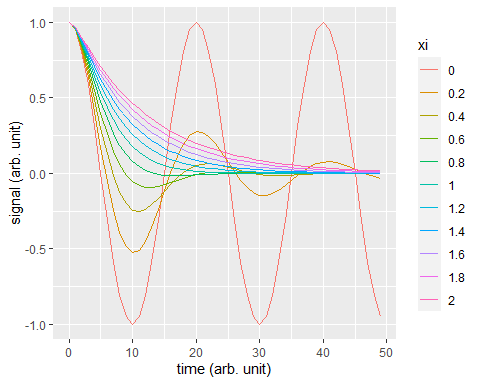## Simulating data

Two functions are available to simulate data in the package: generate.2order simulate the solution of the differential equation for a given vector of time, and the parameters period = $$T$$, xi = $$\xi$$, yeq = $$y_{eq}$$, y0 = $$y(t = 0)$$, v0 = $$\frac{dy}{dt}(t=0)$$, k = $$k$$ and the vector excitation $$u(t)$$. The function create a data.table with a column t for the time, y for the signal, and exc for the excitation.

test <- generate.2order(time = 0:100,y0 = 10,v0 = 0,period = 10,xi = 0.2)
plot(test$t,test$y)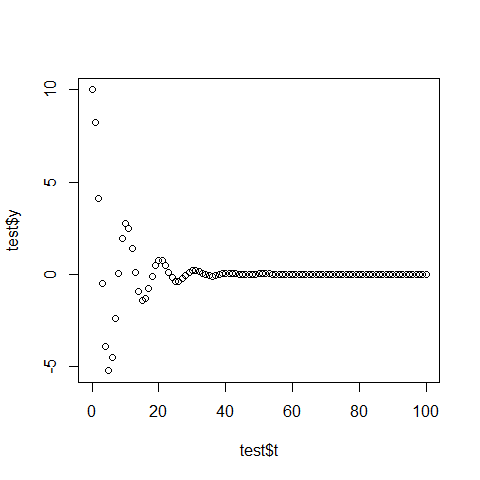The function generate.panel.2order uses generate.2order to generate a panel of nind individuals, with measurement noise and inter-individual noise.

### Example 1 - Generating Damped Linear Oscillator signals with inter-individual variability

The parameter internoise allow the parameters of the differential equation to vary between the individuals. They are in this case distributed along a normal distribution centered on the parameter value given to the generate.panel.2order function with a standard deviation of internoiseparameter. The parameter intranoise allows to ass measurement noise. intranoise is the ratio between the measurement noise’ standard deviation and the signal’ standard deviation.

time <- 0:100
set.seed(123)
data1 <- generate.panel.2order(time = time,
y0 = 10,
xi = 0.1,
period = 30,
yeq = 2,
nind = 6,
internoise = 0.1,
intranoise = 0.3)
data1
#>      id time signalraw     signal
#>   1:  1    0  9.439524 10.0688581
#>   2:  1    1  9.288798  8.8533605
#>   3:  1    2  8.850894 10.1718322
#>   4:  1    3  8.154999  9.4508612
#>   5:  1    4  7.239286  8.4516940
#>  ---
#> 602:  6   96  2.921115 -0.1351427
#> 603:  6   97  2.941171  3.8194867
#> 604:  6   98  2.914748  3.4257195
#> 605:  6   99  2.846029  0.6161688
#> 606:  6  100  2.741759 -0.4584270

When there is no excitation, the input can be set to NULL (default value), like in the example above. As presented on the first order differential equation vignette, the result can be easily plotted through the plot command:

plot(data1) +
ggplot2::geom_hline(yintercept=0)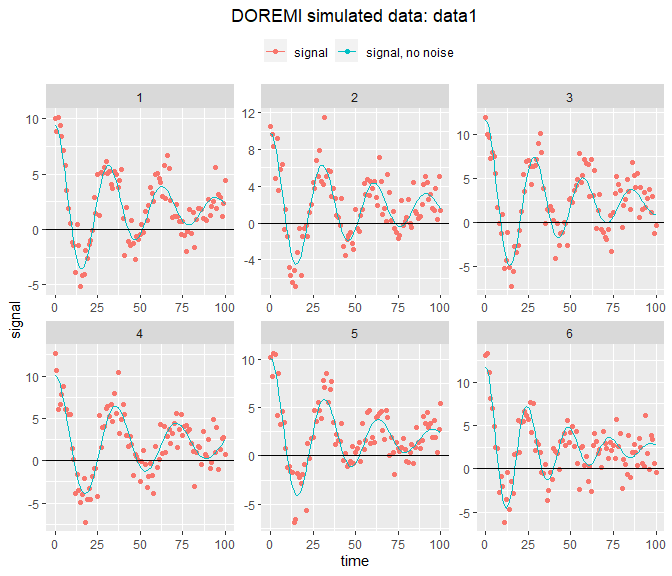We see here that the period, the equilibrium value and the damping parameter vary between each individual.

### Example 2 - Using simulation functions to generate undamped, critically damped and overdamped signals

Let’s note that the damping ratio parameter allows to generate not only oscillating signals, that is when $$0<\xi<1$$, but also signals where the system can reach its equilibrium value without oscillations: these are the critically damped ($$\xi=1$$) and overdamped ($$\xi>1$$). The simulation functions of the package also allow the generation of these behaviors, as shown below:

set.seed(123)
data2a <- generate.panel.2order(time = 0:99,
y0 = 1,
period = 30,
nind = 1,
intranoise = 0.2)
set.seed(123)
data2b <- generate.panel.2order(time = 0:99,
y0 = 1,
xi = 1,
period = 30,
intranoise = 0.2)
set.seed(123)
data2c <- generate.panel.2order(time = 0:99,
y0 = 1,
xi = 2,
period = 30,
intranoise = 0.2)
gridExtra::grid.arrange(plot(data2a)+
ggplot2::ggtitle("undamped, xi=0"),
plot(data2b)+
ggplot2::ggtitle("critically damped, xi=1"),
plot(data2c)+
ggplot2::ggtitle("overdamped, xi=2"), ncol= 3)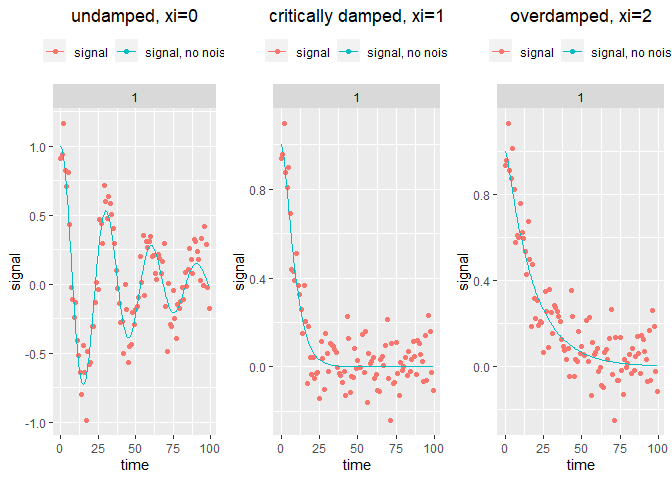## Analyzing data

### Example 1 - Analyzing Damped Linear Oscillator signals

Analyzing the previous dataset and as for the first order model, the user must specify the name of the columns containing the id of the participants, the excitation, and the signal. Several methods are available for the estimation of the derivatives and the user needs to specify which method to use (gold is the default) and the embedding dimension/smoothing parameter (see the package pdf manual for more details).

res1 <- analyze.2order(data = data1,
id = "id",
time ="time",
signal = "signal",
dermethod = "glla",
derparam = 13,
order = 2)
#> WARN [2021-01-19 14:28:41] No excitation signal introduced as input. Input was set to 0.

Now let’s take a look at the result. It is possible to plot the estimated curve from the estimated coefficients, to visually inspect the analysis:

plot(res1)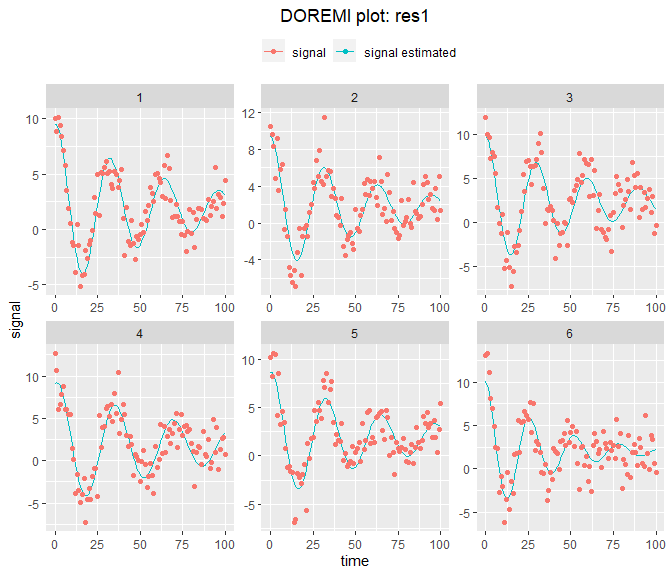The different parts of the resulting doremi object are the same as those for the first order:

• data: contains the original dataset and extra columns for intermediate calculations. The column “signalname_derivate2” is the only additional one with respect to the first order result.

• resultmean: contains the fixed coefficients resulting from the regression for all the individuals. It is also the part of the result displayed when one calls the variable name (that calls the print method for doremi objects):

res1
#>        omega2 omega2_stde   xi2omega esp2omega_stde  yeqomega2 yeqomega2_stde
#> 1: 0.04252023 0.003707686 0.03652068    0.006621061 0.08296443     0.01002565
#>      period        wn        xi      yeq komega2  k        R2
#> 1: 30.47067 0.2062043 0.0885546 1.951176      NA NA 0.7368139

Beware that, as known in the two-steps procedures, and as in the first order case, the estimation of derivatives is a source of bias and thus the error terms provided by the regression are not final. Nevertheless, it is possible to obtain from the summary of the regression the standard errors calculated for the coefficients estimated that will be $$\zeta$$, $$\eta$$ (see equations 3 and 4) and $$y_{eq}\zeta$$ if the equilibrium value is different from 0.

In the resultid object, the first columns are the terms resulting from the regression:

• omega2 is the term $$\omega_n^2$$ or $$\eta$$, with its standard error omega2_stde

• xi2omega is the term $$2\xi \omega_n^2$$ or $$\zeta$$, with its standard error xi2omega_stde

• yeqomega2 is $$y_{eq}\omega_n^2$$ or $$y_{eq}\zeta$$, with its standard error yeqomega2_stde

• komega2 is $$k\omega^2$$ the coefficient associated to the external excitation term in the regression, with its standard error komega2_stde. As there is no excitation on this example, it is NA.

Whereas the following are the values calculated from these first columns:

• period is the oscillation period, that can be extracted from the term $$\omega_n^2$$, were $$T=2\pi/\omega_n$$

• wn is the natural frequency, calculated also from the term omega2 = $$\omega_n^2$$

• xi is the damping factor, calculated from the term xi2omega = $$2\xi \omega_n^2$$

• yeq is the equilibrium value, calculated from yeqomega2 = $$y_{eq}\omega_n^2$$

• k is the gain, calculated from komega2

• resultid: contains the same coefficients but reconstructed for each individual. In the figure above it can be seen that for some of them, the fit wasn’t very good. This will be enhanced using the function optimum_param that will identify which embedding number produces the best estimate, as for the first order case. For each individual we then have:

res1$resultid #> id omega2 xi2omega yeqomega2 period wn xi yeq #> 1: 1 0.03910517 0.03231598 0.07405754 31.77333 0.1977503 0.08170907 1.893804 #> 2: 2 0.04007143 0.03647826 0.06898463 31.38791 0.2001785 0.09111432 1.721541 #> 3: 3 0.04440319 0.03347675 0.10157258 29.81761 0.2107206 0.07943395 2.287506 #> 4: 4 0.03422321 0.02631331 0.06130401 33.96405 0.1849952 0.07111892 1.791300 #> 5: 5 0.03857454 0.03201682 0.07176893 31.99112 0.1964040 0.08150755 1.860526 #> 6: 6 0.05874383 0.05852299 0.12009888 25.92380 0.2423713 0.12073005 2.044451 #> komega2 k #> 1: NA NA #> 2: NA NA #> 3: NA NA #> 4: NA NA #> 5: NA NA #> 6: NA NA • regression: contains the summary of the multilevel regression carried out to fit the coefficients The doremi object will also contain the derivative method used and the embedding number/smoothing parameter used for further reference. # SECOND ORDER DIFFERENTIAL EQUATION MODELS WITH AN EXCITATION TERM ## Simulating data ### Example 1 - Generating signals with no noise In this example we will generate data for 5 individuals, that respond to a “step” excitation (an excitation that changes value abruptly, from 0 to 1 in this case). We will consider no dynamic noise, no variation of the damping factor, period, or equilibrium value across individuals (no interindividual noise). time <- 0:100 data1 <- generate.panel.2order(time = time, excitation = as.numeric(time>20), xi = 0.1, period = 30, k = 1, nind = 5) Plotting once more the data with the plot method available in the package for doremidata objects: plot(data1)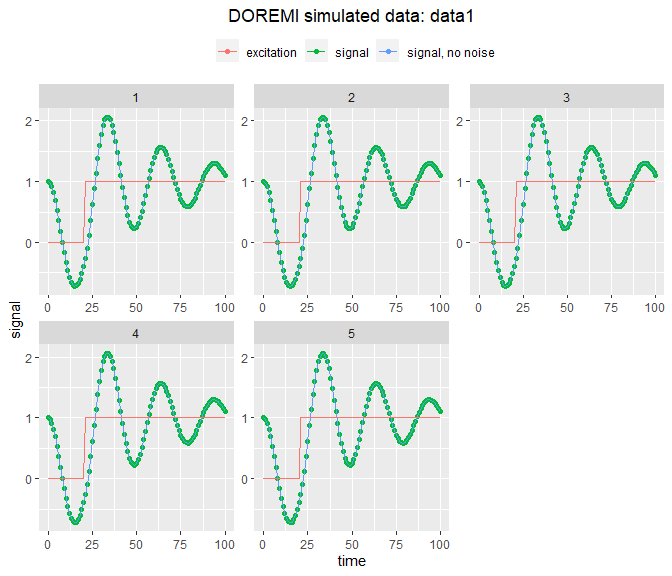### Example 2 - Generating signals with noise The call to the function remains almost the same, this time with a noise to signal ratio of 0.3 and a 20% inter-individual noise: # Generation of signals with intra and inter-noise time <- 0:100 data2 <- generate.panel.2order(time = time, excitation = as.numeric(time>20), xi = 0.1, period = 30, k = 1, nind = 5, internoise = 0.2, intranoise = 0.3) plot(data2)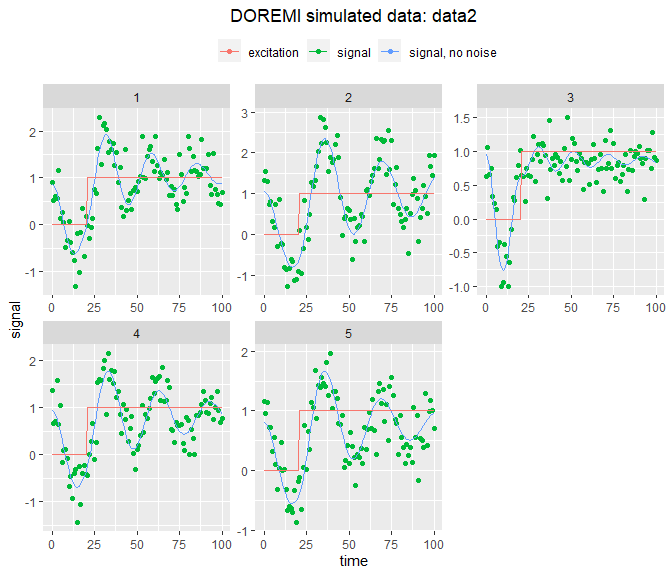And, as it can be seen in the figures, the coefficients change according to the person (damping factor, period, gain). Initial value, speed and equilibrium value could also change if their initial value was different from 0. These have been set to 0 for readability of the results but they could also be included. ### Example 3 - Using an initial condition in a time different from t0=0 The functions to generate the solution of the second order differential equation allow to specify the time for which the initial condition (y0 and v0) are given. This time must be between the minimum and the maximum value of the time vector given to the function. Below an example specifying the value, the derivative of the signal at a given time: time <- 0:99 data3 <- generate.panel.2order(time = time, excitation = as.numeric(time>20), xi = 0.3, period = 30, k = 1, y0 = 2, v0 = 1, t0 = 15,nind = 1) plot(data3)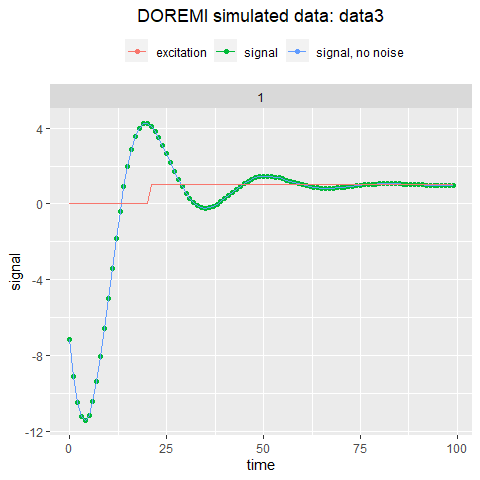The function generate.2order generated for all time given the unique solution of the differential equation that has value 3 at $$t = 25$$ and a derivative of 1 at this point. ### Example 4 - studying the effect of periodical excitations The excitation can be of any form. This package can also be used to simulate driven damped oscillators: t <- 0:99 excitation <- 5*sin(2*pi*t/10) driven_dlo <- generate.panel.2order(time = t, excitation = excitation, y0 = 10, xi = 0.2, period = 20, nind = 1) plot(driven_dlo)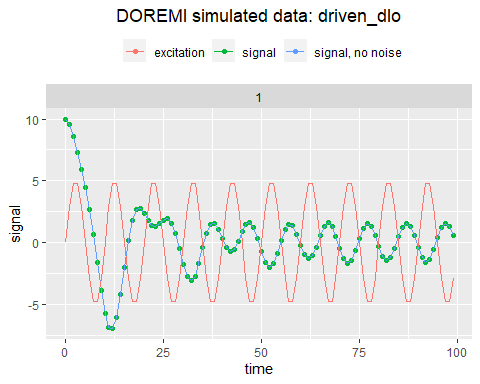Here we can see that the system, which has a natural period of 20, has a steady state that oscillate with the same period as the excitation, that is a period of 10. ## Analyzing data Analyzing the signals generated in the previous examples, we will verify that the parameters were the one introduced in the simulation function and that the estimated signals generated match the simulated ones. ### Example 1 - Analyzing data from a single individual The simplest case is the one in which the data measured corresponds to a single individual. The call to the function is almost the same but omitting the id parameter in the call. The input parameter “verbose” as in other R functions, allows to print (using the package futile.logger) the actions carried out by the function until the calculation of the result: res1 <- analyze.2order(data = data1[id==1], input = "excitation", time ="time", signal = "signal", dermethod = "gold", derparam = 3, verbose=T) #> INFO [2021-01-19 14:28:46] One or several excitations. Linear regression calculated #> INFO [2021-01-19 14:28:46] Linear mixed-effect model had no errors. #> INFO [2021-01-19 14:28:46] Single individual. Calculating gains for one or several excitations #> INFO [2021-01-19 14:28:46] Estimating signal for single individual plot(res1)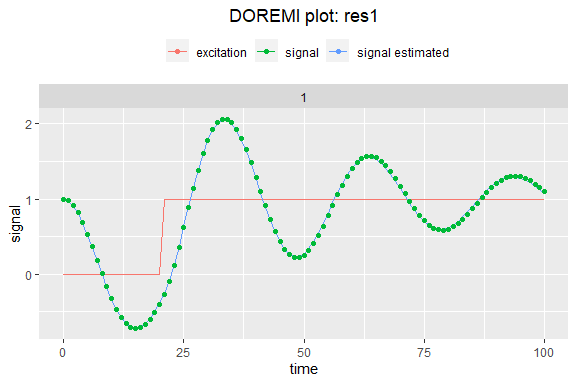### Example 2 - Analyzing data with several individuals and some inter and intra-individual noise Analyzing the data generated in the example 2 of the simulation section, the user must specify the name of the columns containing the id of the participants, the excitation, and the signal. As several methods are available for the estimation of the derivatives, the user needs to specify which method to use (gold is the default) and the embedding dimension (see the package pdf manual for more details). res2 <- analyze.2order(data = data2, id = "id", input ="excitation", time ="time", signal = "signal", dermethod = "gold", derparam = 5, order = 4) plot(res2)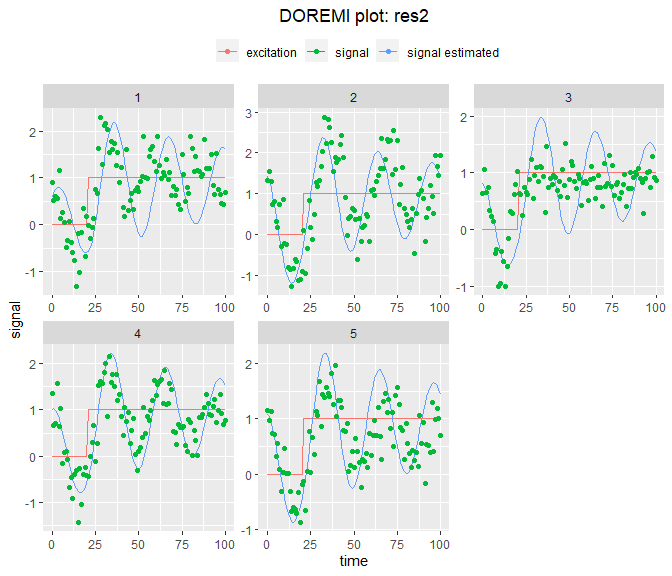### Example 3 - Enhancing the fit by changing the embedding number/ smoothing parameter. As it was mentioned before, the estimation of derivatives is a source of bias. The previous fit can be enhanced in three ways: • By changing the embedding number/smoothing parameter • By changing the order of the derivative • By changing the derivation method In the following example, we will use the function optimum_param of the doremi package to find the embedding number that provides an R2 the closest to 1. The other ways can be tested “manually” by calling the other functions, for instance, in a simulation study. res3 <- optimum_param (data=data2, id="id", input="excitation", time="time", signal="signal", model = "2order", dermethod = "glla", order = 2, pmin = 5, pmax = 17, pstep = 2) #> Warning: Model failed to converge with 2 negative eigenvalues: -4.1e-02 -1.8e+00 #> Warning: Model failed to converge with 1 negative eigenvalue: -9.9e-02 res3$analysis
#>        omega2 omega2_stde   xi2omega esp2omega_stde     yeqomega2
#> 1: 0.15240859 0.017165126 0.07072646    0.050518640 -1.529673e-02
#> 2: 0.08386721 0.010183999 0.05197518    0.026363745 -7.186378e-03
#> 3: 0.06642613 0.011871053 0.04104850    0.016291128 -4.009652e-03
#> 4: 0.05545717 0.010826453 0.03585923    0.010365056 -1.362731e-03
#> 5: 0.04942667 0.009205934 0.03541548    0.008307339 -7.057166e-04
#> 6: 0.04556870 0.008059665 0.03606063    0.007272696 -6.186052e-05
#> 7: 0.04119786 0.006177849 0.04445767    0.006281961  1.671691e-03
#>    yeqomega2_stde   period        wn         xi          yeq excitation_komega2
#> 1:    0.017591424 16.09441 0.3903954 0.09058310 -0.100366572         0.15579155
#> 2:    0.008042482 21.69620 0.2895984 0.08973667 -0.085687571         0.08476531
#> 3:    0.005135935 24.37869 0.2577327 0.07963387 -0.060362571         0.06569553
#> 4:    0.003473488 26.68093 0.2354935 0.07613637 -0.024572668         0.05275712
#> 5:    0.002512501 28.26176 0.2223211 0.07964938 -0.014278052         0.04620178
#> 6:    0.001855513 29.43381 0.2134683 0.08446369 -0.001357522         0.04173378
#> 7:    0.001621059 30.95584 0.2029725 0.10951645  0.040577128         0.03607719
#>    excitation_komega2_stde excitation_k        R2  D
#> 1:             0.025741643    1.0221966 0.2853658  5
#> 2:             0.015358674    1.0107085 0.3187247  7
#> 3:             0.014804667    0.9890014 0.4880996  9
#> 4:             0.012224892    0.9513129 0.7379361 11
#> 5:             0.009979706    0.9347541 0.7653589 13
#> 6:             0.008501695    0.9158432 0.7250433 15
#> 7:             0.006484326    0.8757055 0.6831471 17
res3$summary_opt #> omega2 omega2_stde xi2omega esp2omega_stde yeqomega2 #> 1: 0.04942667 0.009205934 0.03541548 0.008307339 -0.0007057166 #> yeqomega2_stde period wn xi yeq excitation_komega2 #> 1: 0.002512501 28.26176 0.2223211 0.07964938 -0.01427805 0.04620178 #> excitation_komega2_stde excitation_k R2 D method #> 1: 0.009979706 0.9347541 0.7653589 13 glla res3$d
#>  13

And, from the range provided, an embedding number of 13 produces the best fit and that the coefficients are closer to their true values than the ones estimated in the previous example.

If we want to graphically see the evolution of the coefficients according to the embedding number in this case, we can easily plot the results with the plot function. This will call the method for the plotting of “doremiparam” objects:

plot(res3)
#> Warning in [.data.table(analysis, , -c("id")): column(s) not removed because
#> not found: [id]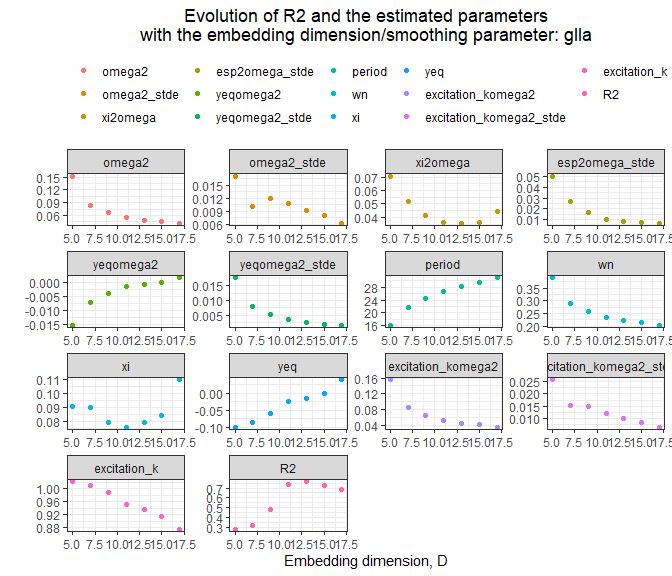And doing again the analysis with the optimum embedding number:

res3b <- analyze.2order(data = data2,
id = "id",
input ="excitation",
time ="time",
signal = "signal",
dermethod = "glla",
derparam = res3$d, order = 2) #> Warning: Model failed to converge with 1 negative eigenvalue: -9.9e-02 res3b #> omega2 omega2_stde xi2omega esp2omega_stde yeqomega2 #> 1: 0.04942667 0.009205934 0.03541548 0.008307339 -0.0007057166 #> yeqomega2_stde period wn xi yeq excitation_komega2 #> 1: 0.002512501 28.26176 0.2223211 0.07964938 -0.01427805 0.04620178 #> excitation_komega2_stde excitation_k R2 #> 1: 0.009979706 0.9347541 0.7653589 plot(res3b)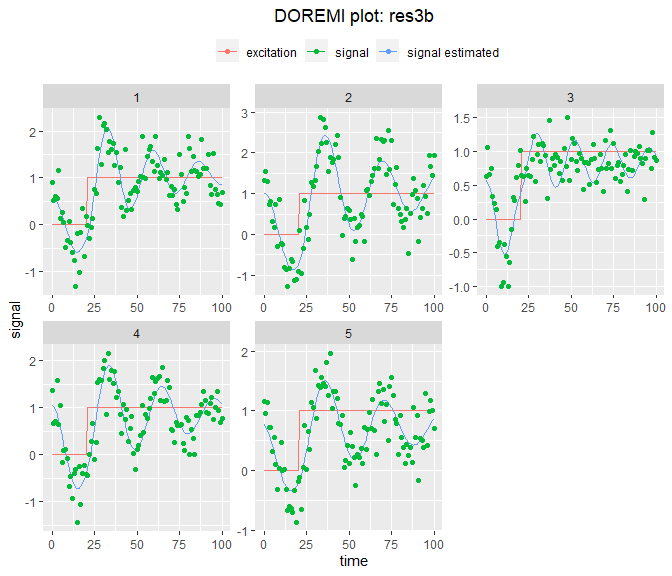### Example 4 - Analyzing data when the signal is subject to several excitations and no noise In this example, we will generate the response to 3 excitations, with a gain different for each excitation. #Simulating data with these hypothesis #Generating the three excitation signals: time <- 1:100 u1 <- as.numeric(time < 20 & time > 10) u2 <- as.numeric(time < 40 & time > 30) u3 <- as.numeric(time < 80 & time > 70) # Arbitrarily choosing a = 1, b = 2 and c = 5 for the first individual et1 <- u1 + 3*u2 + 5*u3 y1 <- generate.2order(time = time, excitation = et1)$y
#as we are using the $y argument of the object generated #Signals for the second individual; # Arbitrarily choosing a = 1, b = 2.5 and c = 4 for the second individual et2 <- u1 + 2.5*u2 + 4*u3 y2 <- generate.2order(time = time, excitation = et2)$y

#Generating table with signals
dataa4 <- data.table::data.table(id = rep(c(1, 2), c(length(et1), length(et2))),
time = c(time, time),
excitation1 = rep(u1,2),
excitation2 = rep(u2,2),
excitation3 = rep(u3,2),
signal_no_noise = c(y1, y2))
dataa4[,signal := signal_no_noise + rnorm(.N,0,0.5)]
dataa4[,excitation := excitation1 + excitation2 + excitation3]
#Plotting signals
ggplot2::ggplot( data = dataa4) +
ggplot2::geom_line(ggplot2::aes(time,signal_no_noise, colour = "Signal_no_noise"))+
ggplot2::geom_point(ggplot2::aes(time,signal, colour = "Signal"))+
ggplot2::geom_line(ggplot2::aes(time,excitation,colour = "Total excitation"))+
ggplot2::facet_wrap(~id)+
ggplot2::labs(x = "Time (s)",
y = "Signal (arb. unit)",
colour = "")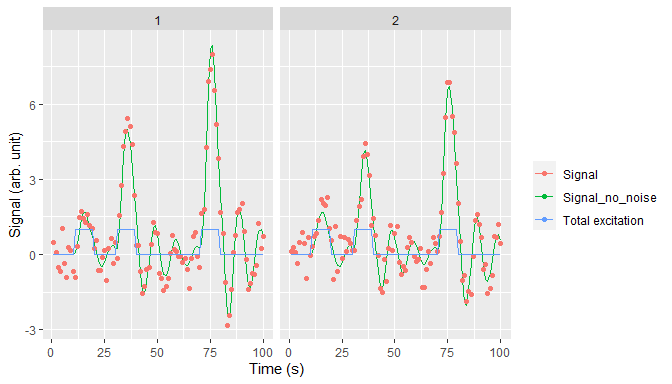We see that we generate three different amplitudes of response for these three excitations. It is possible to estimate the gain for each excitation by giving a vector of the different excitation column to the analyze.2order function, as shown below:

#Analyzing signals
res4 <- analyze.2order(data = dataa4,
id = "id",
input = c("excitation1", "excitation2", "excitation3"),
time = "time",
signal = "signal",
dermethod = "glla",
derparam = 7)

#Looking for the calculation of the coefficients of the excitation
res4
#>       omega2 omega2_stde  xi2omega esp2omega_stde  yeqomega2 yeqomega2_stde
#> 1: 0.2856476 0.007940104 0.1095049     0.01598097 0.01674109     0.01437996
#>      period        wn        xi        yeq excitation1_komega2
#> 1: 11.75613 0.5344601 0.1024444 0.05860748           0.2821087
#>    excitation1_komega2_stde excitation1_k excitation2_komega2
#> 1:               0.04120383     0.9876106           0.7429894
#>    excitation2_komega2_stde excitation2_k excitation3_komega2
#> 1:                0.1197821      2.601069            1.179005
#>    excitation3_komega2_stde excitation3_k        R2
#> 1:                0.1120865      4.127481 0.7629952
res4$resultid #> id omega2 xi2omega yeqomega2 period wn xi yeq #> 1: 1 0.2880801 0.1005757 0.005159723 11.70640 0.5367309 0.0936929 0.01791073 #> 2: 2 0.2832152 0.1184341 0.028322456 11.80651 0.5321797 0.1112726 0.10000329 #> excitation1_komega2 excitation1_k excitation2_komega2 excitation2_k #> 1: 0.2513599 0.872535 0.8546760 2.966800 #> 2: 0.3128574 1.104663 0.6313028 2.229057 #> excitation3_komega2 excitation3_k #> 1: 1.278386 4.437606 #> 2: 1.079625 3.812030 And one can find the gains estimated for each excitation by extracting them from the $resultid. They are a good approximation of the coefficients introduced.

#Plotting signals
plot(res4)